Quick guide for analyzing the multitrait multimethod  structural equation modeling approach to construct validity.

Merle Canfield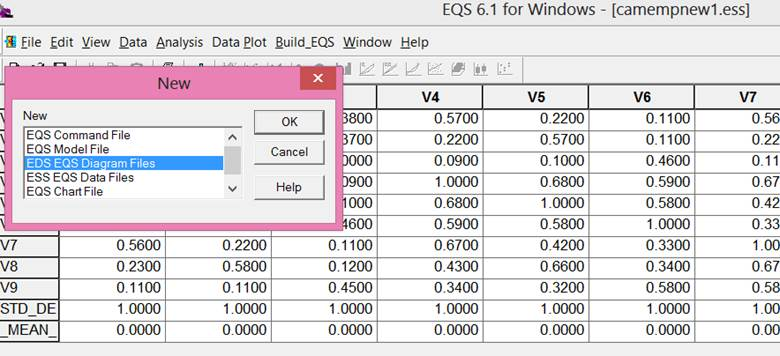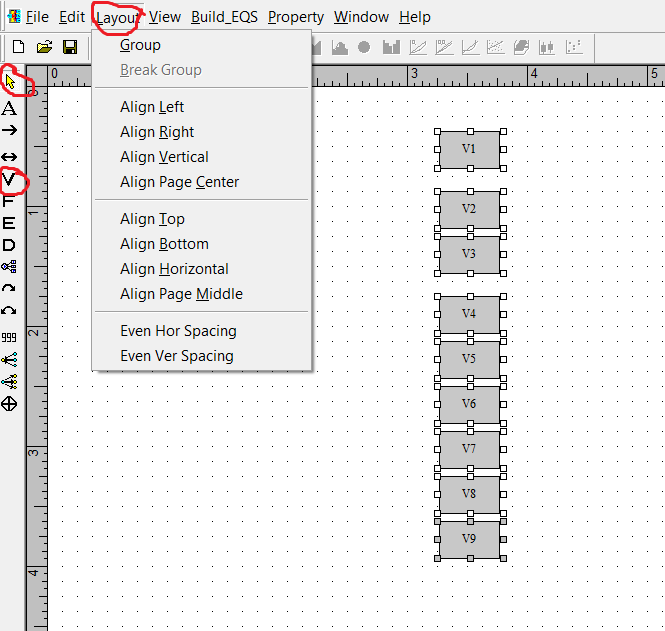First make the column of variables by clicking on the V (circled in red).  Then start at the top and click once for each variable.  You can’t quite get them aligned exactly – just try to get close.  Then use the yellow arrow to select all of the variables.  Then click Layout (circled in red) and click on Align Left and then Even Ver Spacing.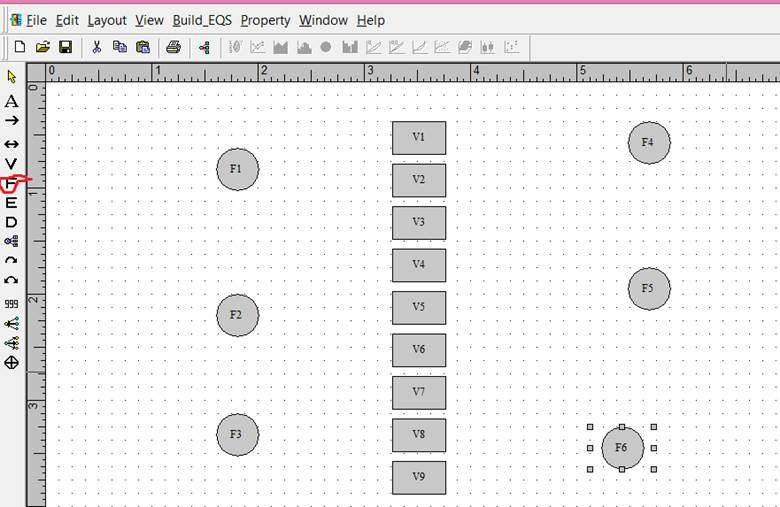The factors were generated by clicking on the F (circled in red). Each column of factors is given the same treatment with Layout as was given to variables to align them.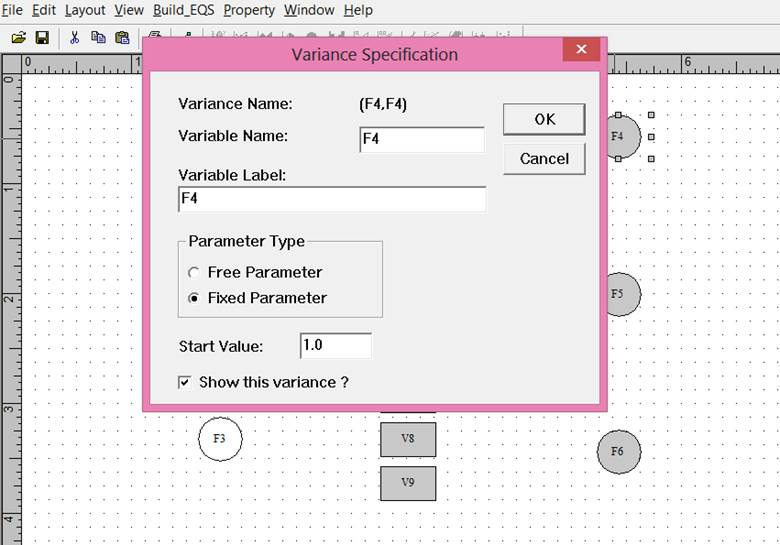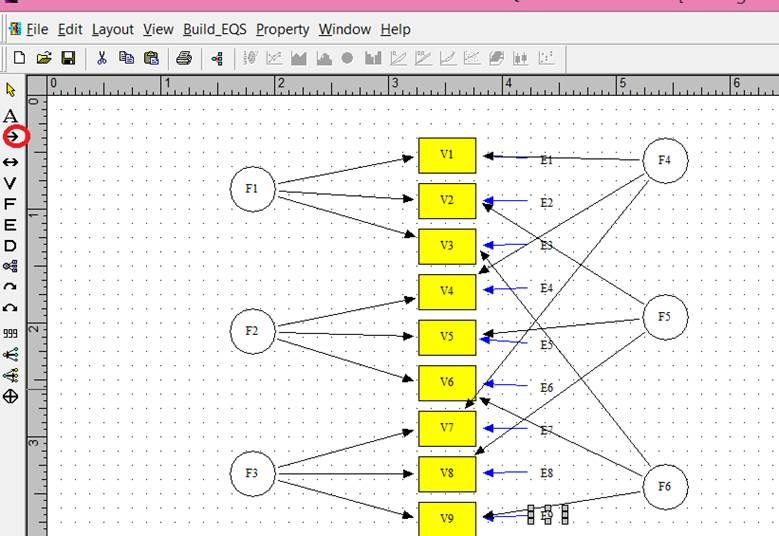The parameters from the factors to the variables are generated by clicking on the straight arrow (circled in red) and then clicking within the factor and then the desired variable.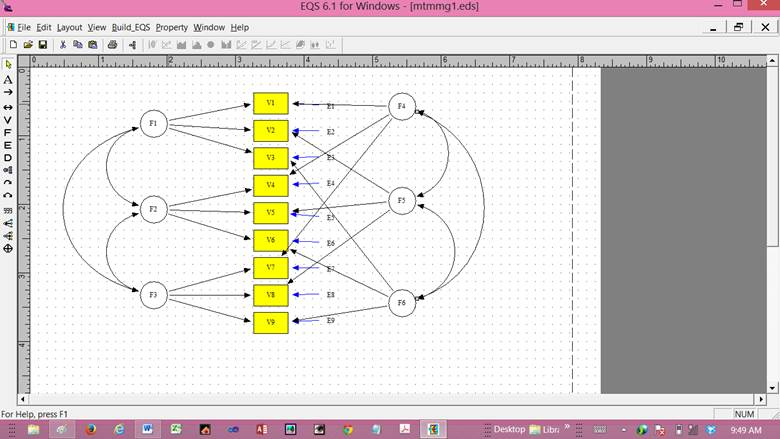Model 1.  Full model.

Four models are required.           The procedure described by Byrne (2010) involves four different analyses. The analyses are: (1) the full model as presented in Figure 1; (2) methods only (all trait factors and corresponding parameters were removed and the method factors allowed to correlate) (model 2);  (3) all of the correlations among the trait factors were set to 1.00 and the method factors allowed to correlate (model 3); and (4) the correlation between the method factors was eliminated and the trait factors allowed to correlate (model 4). The results from these four analyses are in Table 2.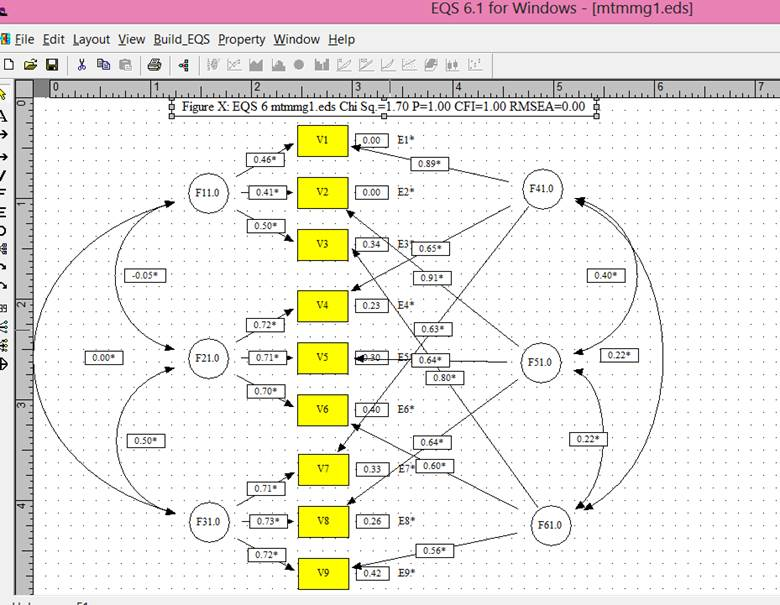Model 1.  Full model.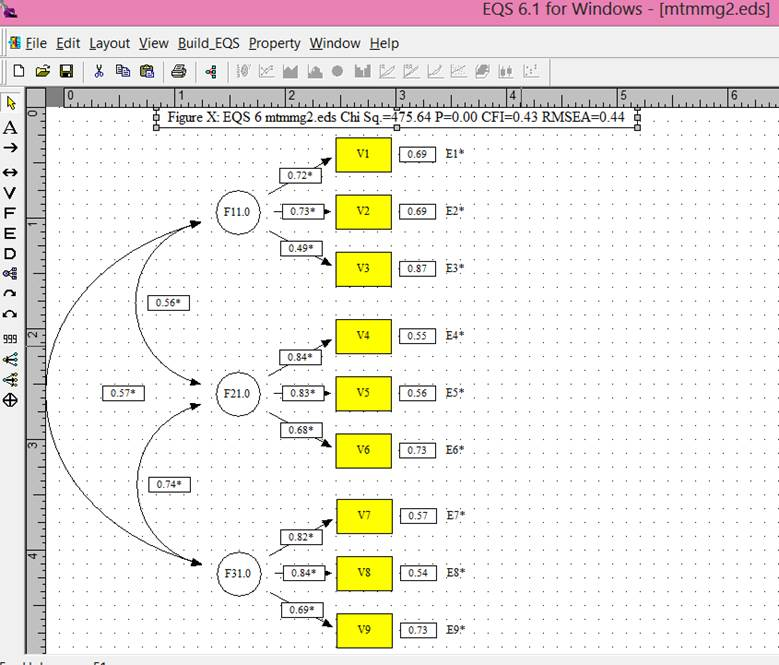Model 2.  Traits eliminated.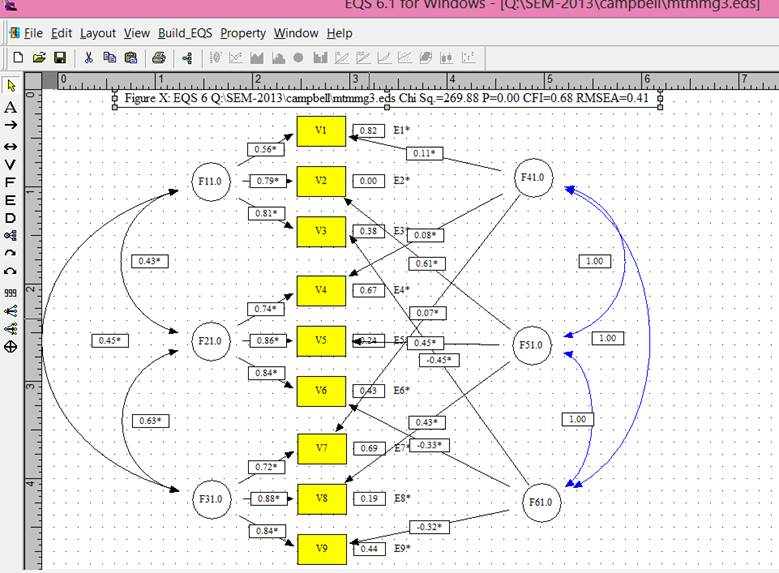Model 3.  Trait factor correlations contrained to 1.0.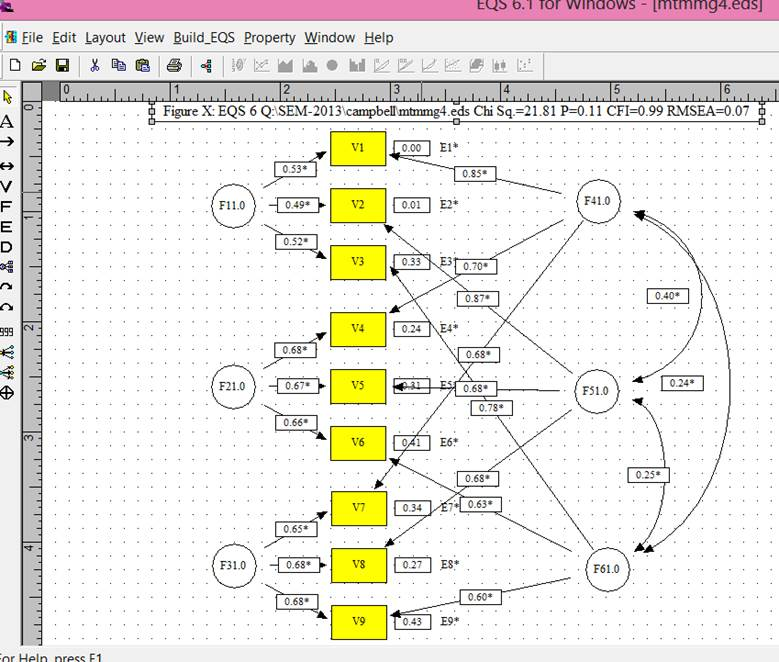Model 4.  Method factors not allowed to correlate.

Table 2.  The c2, degrees of freedom, and comparative fit index for each of the four disciplinemodels

 Model 1 Model 2 Model 3 Model 4 c2 1.969 475.636 269.884 21.813 df 12 24 15 15 cfi 1.00 0.429 0.678 0.991

Convergent and discriminant validity are assessed by two different methods: one method uses the c2,the other the comparative fit indexes. Both methods included differences among these indexes in the four models. Table 3 shows the differences among the models and which kind of validity is assessed by the difference. 19.844

Table 3.  Comparison of goodness-of-fit of the three discipline models

 c2 df cfi model 1 vs model 2 473.667** 12 0.571 model 1 vs model 3 267.915** 3 0.322 model 1 vs model 4 19.844** 3 0.009

** indicates significance at .01 level of probability

The significant difference between model 1 and model 2 as evidenced by the significant c2 (473.667 with 12 df and p<.01) and the comparative fit index greater than .01 is indicative of convergent validity. The significant difference between model 1 and model 3 as evidenced by the significant c2 (267.915 with 3 df and p<.01) and the comparative fit index greater than .01 is indicative of discriminant validity. Also indicative of discriminant validity is the lack of significant difference between model 1 and model 4 (c2 = 19.844 with 3 df results in a p<.01).

Convergent validity is supported by the significant c2 difference between model 1 and model 2 indicated and the CFI change greater than .01. Discriminant validity is supported by the significant c2 of 473.667 between model 1 and model 3 and the CFI of .571 and is further supported by the non-significant c2 of 19.844 between model 1 and model 4 and the CFI of 0.001.